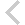FICO Xpress Optimization Examples Repository
 FICO Optimization Community FICO Xpress Optimization HomeModeling with user functions

Description
Shows how to create and solve a nonlinear problem whose constraints and objective functions are defined as user functions

Further explanation of this example: 'Xpress Python Reference Manual'

Source Files
By clicking on a file name, a preview is opened at the bottom of this page.

example_userfunc.py

# Python equivalent of the ComplexUserFunction.c example in
# examples/nonlinear/c directory
#
# (C) Fair Isaac Corp., 1983-2021

# Define objective and constraint as user functions that return
# derivatives
#
# Minimize myobj (y,z,v,w)
#   s.t.
#   ball (x,t) <= 730
#   1 <= x <= 2
#   2 <= y <= 3
#   3 <= z <= 4
#   4 <= v <= 5
#   5 <= w <= 6
#
# where
#
# myobj (y,z,v,w) = y**2 + z - v + w**2
# ball  (x,t) = x**2 + t**2

import xpress

def myobj(y, z, v, w):
'''Return value of the objective function with the derivative w.r.t. all variables passed
'''
return (y**2 + z - v + w**2,  # value of the function
2*y,  # derivative w.r.t. y
1,    # derivative w.r.t. z
-1,   # derivative w.r.t. v
2*w)  # derivative w.r.t. w

def ball(x, t):
'''Return value of the left-hand side of the constraint with its derivatives
'''
return (x**2 + t**2,
2*x,
2*t)

def myobj_noderiv(y, z, v, w):
'''Return objective without derivatives
'''
return (y**2 + z - v + w**2)  # value of the function

def ball_noderiv(x, t):
'''Return left-hand side of constraint without derivatives
'''
return (x**2 + t**2)

x = xpress.var(lb=1, ub=2)
y = xpress.var(lb=2, ub=3)
z = xpress.var(lb=3, ub=4)
v = xpress.var(lb=4, ub=5)
w = xpress.var(lb=5, ub=6)

t = xpress.var(lb=-xpress.infinity)  # free variable

p = xpress.problem()

p.addVariable(x, y, z, v, w, t)

p.setObjective(t)
p.addConstraint(t == xpress.user(myobj, y, z, v, w, derivatives=True))
p.addConstraint(xpress.user(ball, x, t, derivatives=True) <= 730)

p.solve()

print('Problem solved using derivatives. Objective:', p.getObjVal(), '; solution:', p.getSolution())

p = xpress.problem()

p.addVariable(x, y, z, v, w, t)

p.setObjective(t)
p.addConstraint(t == xpress.user(myobj_noderiv, y, z, v, w))

p.solve()

print('Problem solved without derivatives. Objective:', p.getObjVal(), '; solution:', p.getSolution())

p = xpress.problem()

p.addVariable(x, y, z, v, w, t)

p.setObjective(t)
p.addConstraint(t == xpress.user(myobj_noderiv, y, z, v, w))
p.addConstraint(xpress.user(ball, x, t, derivatives=True) <= 730)

p.solve()

print('Problem solved with a mixed setting. Objective:', p.getObjVal(), '; solution:', p.getSolution())